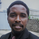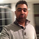# Stochastic + RSI, Double Strategy (by ChartArt)

28766 뷰
28766
This strategy combines the classic RSI strategy to sell when the RSI increases over 70 (or to buy when it falls below 30), with the classic Stochastic Slow strategy to sell when the Stochastic oscillator exceeds the value of 80 (and to buy when this value is below 20).

This simple strategy only triggers when both the RSI and the Stochastic are together in a overbought or oversold condition. The one hour chart of the S&P 500 worked quite well recently with this double strategy.

By the way this strategy should not be confused with the 'Stochastic RSI', which measures the RSI only.

All trading involves high risk; past performance is not necessarily indicative of future results.
```//@version=2
strategy("Stochastic + RSI, Double Strategy (by ChartArt)", shorttitle="CA_-_RSI_Stoch_Strat", overlay=true)

// ChartArt's Stochastic Slow + Relative Strength Index, Double Strategy
//
// Version 1.0
// Idea by ChartArt on October 23, 2015.
//
// This strategy combines the classic RSI
// strategy to sell when the RSI increases
// over 70 (or to buy when it falls below 30),
// with the classic Stochastic Slow strategy
// to sell when the Stochastic oscillator
// exceeds the value of 80 (and to buy when
// this value is below 20).
//
// This simple strategy only triggers when
// both the RSI and the Stochastic are together
// in overbought or oversold conditions.
//
// List of my work:

///////////// Stochastic Slow
Stochlength = input(14, minval=1, title="lookback length of Stochastic")
StochOverBought = input(80, title="Stochastic overbought condition")
StochOverSold = input(20, title="Stochastic oversold condition")
smoothK = input(3, title="smoothing of Stochastic %K ")
smoothD = input(3, title="moving average of Stochastic %K")
k = sma(stoch(close, high, low, Stochlength), smoothK)
d = sma(k, smoothD)

///////////// RSI
RSIlength = input( 14, minval=1 , title="lookback length of RSI")
RSIOverBought = input( 70  , title="RSI overbought condition")
RSIOverSold = input( 30  , title="RSI oversold condition")
RSIprice = close
vrsi = rsi(RSIprice, RSIlength)

///////////// Double strategy: RSI strategy + Stochastic strategy

if (not na(k) and not na(d))
if (crossover(k,d) and k < StochOverSold)
if (not na(vrsi)) and (crossover(vrsi, RSIOverSold))
strategy.entry("LONG", strategy.long, comment="StochLE + RsiLE")

if (crossunder(k,d) and k > StochOverBought)
if (crossunder(vrsi, RSIOverBought))
strategy.entry("SHORT", strategy.short, comment="StochSE + RsiSE")

//plot(strategy.equity, title="equity", color=red, linewidth=2, style=areabr)```Hello,
I'm new to trading and MT4. Please can some one be kind enough to explain how I can compile this script on MQL.
Thank you
응답This is exactly what I've been looking for - however, I am noticing that your script is activating on the stochastics cross but not looking at RSI. Isn't it meant to give signal only when both the Stoch + RSI are either overbought or oversold together?
응답While i'm very new to understanding PineScript, in your explanation of the strategy it seems as if you state it will trigger a buy when both targets have been met RSI falls below 30 and STO gets a KD cross below 20. In your script it seems as if RSI has to cross back above 30 to signal a buy? Also curious as when i drop RSI 14 and STO 14-3-3 below the chart the buys and sells that appear as an overlay to the chart don't quite seem to follow the rules? What i mean by that is an example of the buys and sells that take place from 11/11/15 23:00 to 11/20/15 16:00 . There are times where STO is crossing in oversold territory but RSI>30 (not having been recently oversold) and a buy will occur. What am I missing. Any insight you can provide would be helpful, i've been fiddling with a very similar approach and am interested in what you are doing.
응답